﻿ 基于气候弹性模型的丹江口水库水源区径流模拟及预测
 期刊检索： 题目 作者 关键词
 暴雨灾害2018, Vol. 37Issue (2): 174-180.  DOI: 10.3969/j.issn.1004-9045.2018.02.009DOI

10.3969/j.issn.1004-9045.2018.02.009

资助项目

2016年中国气象局气候变化专项(CCSF201620)；湖北电力公司科技项目(SGTYHT/16-YJ-179)；气象关键技术集成与应用项目(CMAGJ2014M35)

文章历史

1. 国网湖北省电力公司电力科学研究院, 武汉 430077;
2. 国家电网公司高压电器设备现场实验技术重点实验室，武汉 430077;
3. 武汉区域气候中心，武汉 430074

Simulation and forecasting of inflow in the water source area of Danjiangkou reservoir based on the climate elasticity model
, WANG Miao3, GAO Zhengxu3, QIN Pengcheng3, REN Yongjian3
1. State Grid Hubei Electric Power Research Institute, Wuhan 40077;
2. State Grid Key Laboratory of On-site Test Technology on High Voltage Power Apparatus, Wuhan 40077;
3. Wuhan Regional Climate Center, Wuhan 430074
Abstract: Using datasets of diurnal precipitation and temperature at 41 meteorological stations in the water source area of Danjiangkou reservoir as well as the inflow data from this reservoir during 1961-2015, we have analyzed the hydrometeorological characteristics in the water source area of this reservoir for establishing a climate elasticity fitting formula to simulate the inflow series, and then conducted forecasts for the future runoff evolution based on the estimations of precipitation and temperature under three emission scenarios of RCP2.6, RCP 4.5 and RCP 8.5. The results indicate that the temperature in the water source area exhibited a significantly upward trend at 0.13℃ per decade from 1961 to 2015, and its increase mainly appeared after 1990s'. There are clear interannual and interdecadal variations of inflow over the past 50 years, whose decreasing trend is overall 64.3 m3·s-1 per decade. The variation tendency of inflow can commendably be simulated by the climate elasticity model, and the elasticity coefficient of runoff to precipitation is higher than to temperature. Both precipitation and temperature are in increasing trend and inflow shows different tendencies under the three emission scenarios of RCP2.6, RCP 4.5 and RCP 8.5. In RCP2.6 emissions scenario, the interdecadal inflows are in decreasing trend, whose decrement exceeded 270 m3·s-1. In RCP4.5 emissions scenario, the interdecadal inflows all are in increasing trend. In RCP8.5 emissions scenario, the interannual inflows fluctuate severely and decrease by -14.2 m3·s-1 per decade, which meant that it would not be favorable to the managing of water resources.
Key words: inflow    precipitation    temperature    climate elasticity model    Danjiangkou reservoir

1 资料与方法 1.1 资料说明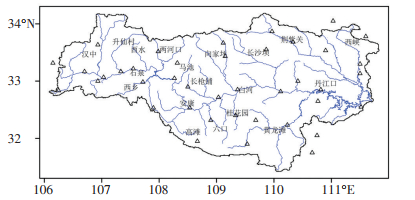图 1 丹江口水库水源区地理位置与气象站点(空心三角形所示)分布 Fig. 1 The water source area geographical position of Danjiangkou reservoir and spatial distribution of weather stations (showed by hollow triangles) over this area.
1.2 气候变化弹性模型的建立方法

 $\varepsilon = \frac{{{\rm{d}}Q/Q}}{{{\rm{d}}X/X}}$ (1)

 $\varepsilon = \frac{{\Delta {Q_i}/\bar Q}}{{\Delta {X_i}/\bar X}}$ (2)

 $\Delta {Q_i}/\bar Q = \varepsilon \Delta {X_i}/\bar X$ (3)

 $\varepsilon = \frac{{\bar X}}{{\bar Q}}\frac{{\sum {\left( {{X_i}/\bar X} \right)\left( {{Q_i}/\bar Q} \right)} }}{{\sum {{{\left( {{X_i}/\bar X} \right)}^2}} }} = {\rho _{Q - X}}{C_Q}/{C_X}$ (4)

 ${\rho _{Q - X}} = \frac{{\sum {\left( {{Q_i}/\bar Q} \right)\left( {{X_i}/\bar X} \right)} }}{{\sqrt {\sum {{{\left( {{X_i}/\bar X} \right)}^2}} .\sum {{{\left( {{Q_i}/\bar Q} \right)}^2}} } }}$ (5)
 ${C_Q} = \frac{{\sqrt {\frac{{\sum {{{\left( {{Q_i}/\bar Q} \right)}^2}} }}{n}} }}{{\bar Q}}$ (6)
 ${C_X} = \frac{{\sqrt {\frac{{\sum {{{\left( {{X_i}/\bar X} \right)}^2}} }}{n}} }}{{\bar X}}$ (7)

 $\Delta {Q_i}/\bar Q = {\varepsilon _P} \cdot \Delta {P_i}/\bar P + {\varepsilon _t} \cdot \Delta {T_i}/\bar T$ (8)

 ${Q_i} = \bar Q + \Delta {Q_i}$ (9)

2 丹江口水库入库径流变化特征分析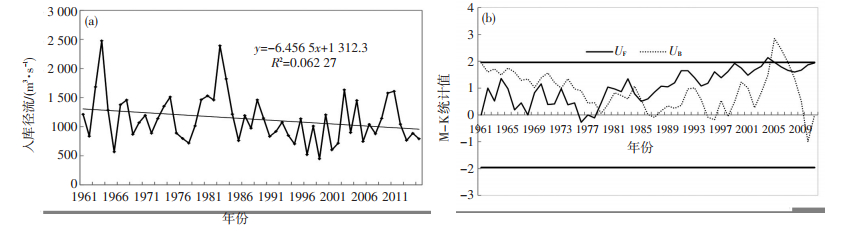图 2 1961—2015年丹江口水库入库径流(a，单位: m3·s-1)的逐年变化与M-K突变检验(b) Fig. 2 (a) Annual variation and (b) M-K mutation test for the inflow (unit: m3·s-1) of Danjiangkou reservoir during 1961-2015.
3 不同时期丹江口水库水源区气候弹性模型建立与适用性分析 3.1 基准期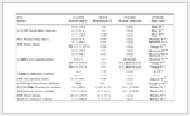表 1 丹江口水库水源区基准期(1961—1985年)和变化期(1986—2015年)径流及相关要素值 Table 1 Annual runoff and related factors between Basic Period (1961-1985) and Change Period (1986-2015) in the water source area of Danjiangkou reservoir.

 $\begin{array}{l} {Q_i} = 1288.7 + 1288.7 \cdot \left( {0.92 \cdot \frac{{\left( {{P_i} - 873.8} \right)}}{{873.8}}} \right) + \\ \;\;\;\;\;\;\left. {0.60 \cdot \frac{{\left( {{T_i} - 14} \right)}}{{14}}} \right) \end{array}$ (10)

 ${R^2} = \frac{{{{\left[ {\sum\limits_{i = 1}^n {\left( {{Q_{\rm{o}}} - {Q_{{\rm{avg}}}}} \right)\left( {{Q_i} - {Q_{{\rm{avg}},i}}} \right)} } \right]}^2}}}{{\sum\limits_{i = 1}^n {{{\left( {{Q_{\rm{o}}} - {Q_{{\rm{avg}}}}} \right)}^2}} \sum\limits_{i = 1}^n {{{\left( {{Q_i} - {Q_{{\rm{avg}},i}}} \right)}^2}} }}$ (11)
 ${E_{{\rm{ns}}}} = 1 - \frac{{\sum\limits_{i = }^n {{{\left( {{Q_{\rm{o}}} - {Q_p}} \right)}^2}} }}{{\sum\limits_{i = 1}^n {{{\left( {{Q_{\rm{o}}} - {Q_{{\rm{avg}}}}} \right)}^2}} }}$ (12)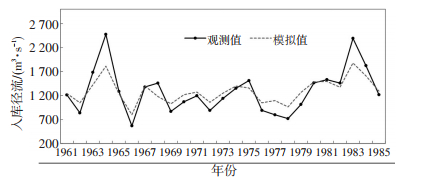图 3 基于气候弹性模型的丹江口水库基准期(1961—1985年)入库径流的模拟与观测序列 Fig. 3 Observed inflow and the inflow of Danjiangkou reservoir simulated based on the climate elasticity model in the Basic Period (1961-1985).
3.2 变化期

 $\begin{array}{l} {Q_i} = 1000.5 + 1000.5 \cdot \left( {0.86 \cdot \frac{{\left( {{P_i} - 835.6} \right)}}{{835.6}}} \right) + \\ \;\;\;\;\;\;0.56 \cdot \frac{{\left( {{T_i} - 14.3} \right)}}{{14.3}} \end{array}$ (13)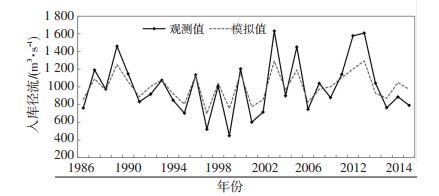图 4 基于气候弹性模型的丹江口水库变化期(1986—2015年)入库径流的模拟与观测序列 Fig. 4 Same as Fig. 3 but for in the Change Period (1986-2015).
4 径流模拟结果与未来情景下径流变化预估 4.1 模拟结果分析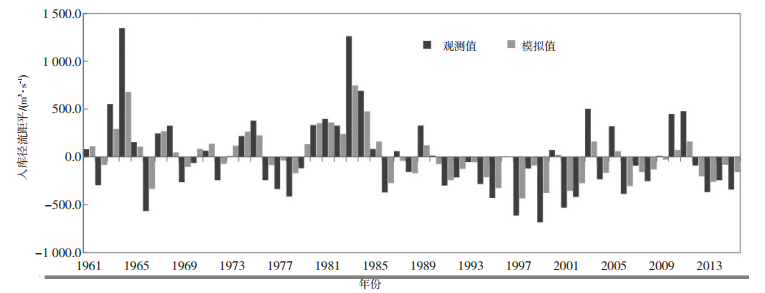图 5 基于气候弹性模型建立的1961—2015年丹江口水库入库径流距平的模拟与观测序列 Fig. 5 Inflow anomalies observed and the inflow anomalies of Danjiangkou reservoir simulated based on the climate elasticity model dated from 1961 to 2015.
4.2 未来情景下径流变化预估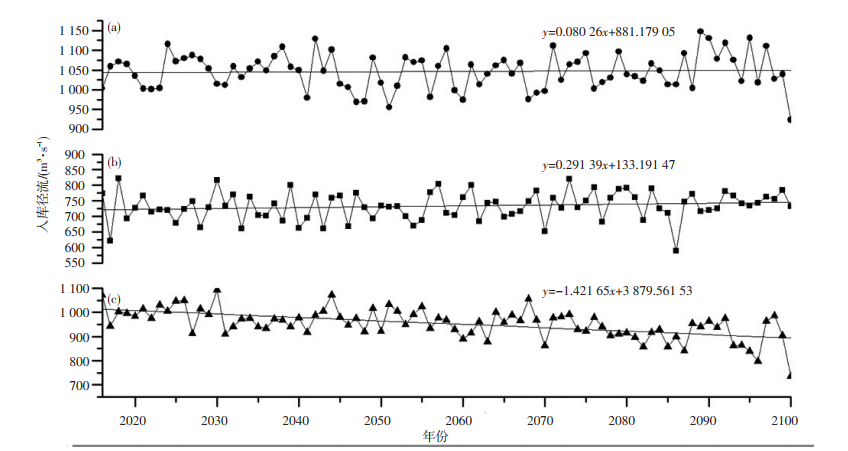图 6 不同未来情景下(a. RCP4.5；b. RCP2.6；c. RCP8.5) 2016—2100年丹江口水库水源区径流模拟序列 Fig. 6 Annual variations of simulated inflow in the water source area of Danjiangkou reservoir dated from 2016 to 2100 in the three emissions scenarios of (a) RCP4.5, (b) RCP2.6 and (c) RCP8.5.表 2 不同未来情景下(RCP2.6、RCP4.5、RCP 8.5)各年代丹江口水库水源区降水、气温及径流变化 Table 2 Interdecadal variations of precipitation, temperature and inflow in the water source area of Danjiangkou reservoir in the three emissions scenarios of RCP2.6, RCP4.5 and RCP8.5.
5 结论与讨论

(1) 丹江口水库水源区近50多年来降水呈微弱减少趋势，气温以0.13 ℃·(10 a)-1的速率呈显著增加，增温主要发生在1990年代以后，入库径流以-64.3 m3·s-1·(10 a)-1的速率呈减少趋势，1960—1980年代为入库径流偏多期，1990年代以来为偏少期，21世纪以来入库径流又趋于增加。

(2) 水库入库径流与降水呈正相关，与气温呈负相关，降水对入库径流影响更明显；径流对降水和气温的弹性系数在1961—1985年期间分别为1.04、-1.02，在1986—2015年期间分别为1.05、-0.75，基于气候弹性模型建立公式，开展的径流模拟能很好地呈现水库入库径流演变特征。

(3) 未来RCP2.6、RCP4.5情景下径流量分别呈0.8 m3·s-1·(10 a)-1、2.91 m3·s-1· (10 a)-1的增加趋势，RCP8.5情景下则呈现-14.2 m3·s-1· (10 a)-1的减少趋势，且RCP8.5情景下年际间波动最剧烈，不利于水资源调度。

  陈杨. 基于ArcGISDesktop丹江口入库径流分析[D]. 武汉: 华中科技大学, 2010 http://www.wanfangdata.com.cn/details/detail.do?_type=degree&id=D139941  郭海晋, 金蓉玲. 丹江口水库上游水资源现状及变化趋势[J]. 自然资源, 1997, 34(1): 28-33.  陆华友, 邵东国, 郭元裕. 丹江口水库径流长期预报研究[J]. 武汉水利电力大学学报, 1996, 29(6): 6-10.  刘明宽, 徐元顺, 朱理国, 等. 丹江口水库流域致洪过程的水文气候特征分析[J]. 暴雨灾害, 2001, 20(4): 15-16.  李英, 李致家, 张利升, 等. 丹江口水库北调水量的不确定性分析[J]. 武汉大学学报(工学版), 2008, 41(3): 34-37.  李凌程, 张利平, 夏军, 等. 气候波动和人类活动对南水北调中线工程典型流域径流影响的定量评估[J]. 气候变化研究进展, 2014, 10(2): 118-126.  徐翔宇. 气候变化下典型流域的水文响应研究[D]. 北京: 清华大学, 2012 http://cdmd.cnki.com.cn/Article/CDMD-10003-1013016560.htm  杨大文, 李翀, 倪广恒, 等. 分布式水文模型在黄河流域的应用[J]. 地理学报, 2004, 59(1): 143-154. DOI:10.11821/xb200401018  王苗, 刘敏, 夏智宏. 基于SWAT模型未来气候变化对洪湖流域水资源影响研究[J]. 气象与环境学报, 2016, 32(4): 39-47.  夏智宏, 刘敏, 王苗, 等. 1990s以来气候变化和人类活动对洪湖流域径流影响的定量辨识[J]. 湖泊科学, 2014, 26(4): 515-521. DOI:10.18307/2014.0404  祝诗学, 梁忠民, 戴昌军, 等. 丹江口水库流域月尺度降雨与径流预报研究[J]. 南水北调与水利科技, 2016, 14(1): 96-101.  Schaake J C. From climate to flow, in Climate Chang and U S Water Resources chap 8, edited by Waggoner P E[M]. New York: John Wiley, 1990, 177-206.  Dooge J C I. Sensitivity of Runoff to Climate Change: A Hortonian Approach[J]. Bulletin of the American Meteorological Society, 1992, 73(12): 2003-2013.  Fu G, Charles S P, Chiew F H S. A two-parameter climate elasticity of streamflow index to assess climate change effects on annual streamflow[J]. Water Resources Research, 2007, 43(11): 2578-2584.  Ma H, Yang D W, Tan S K, et al. Impact of climate variability and human activity on streamflow decrease in the Miyun Reservoir catchment[J]. Journal of Hydrology, 2010, 389(3): 317-324.  陈莹, 陈兴伟. 基于弹性分析的闽江流域径流演变与气候变化关系[J]. 福建师范大学学报(自然科学版), 2011, 27(6): 101-105.  杨娜, 赵巧华, 闫桂霞, 等. 气候变化和人类活动对丹江口入库径流的影响及评估[J]. 长江流域资源与环境, 2016, 25(7): 1129-1134.  王元超, 王旭, 雷晓辉, 等. 丹江口水库入库径流特征及其演变规律[J]. 南水北调与水利科技, 2015, 13(1): 15-19.  盛春淑, 罗定贵. 基于AVSWAT丰乐河流域水文预测[J]. 中国农学通报, 2006, 22(9): 493-496.  郭晓军, 崔鹏, 朱兴华. 典型泥石流流域蒋家沟的降雨-径流模拟[J]. 水土保持通报, 2011, 31(1): 175-179.